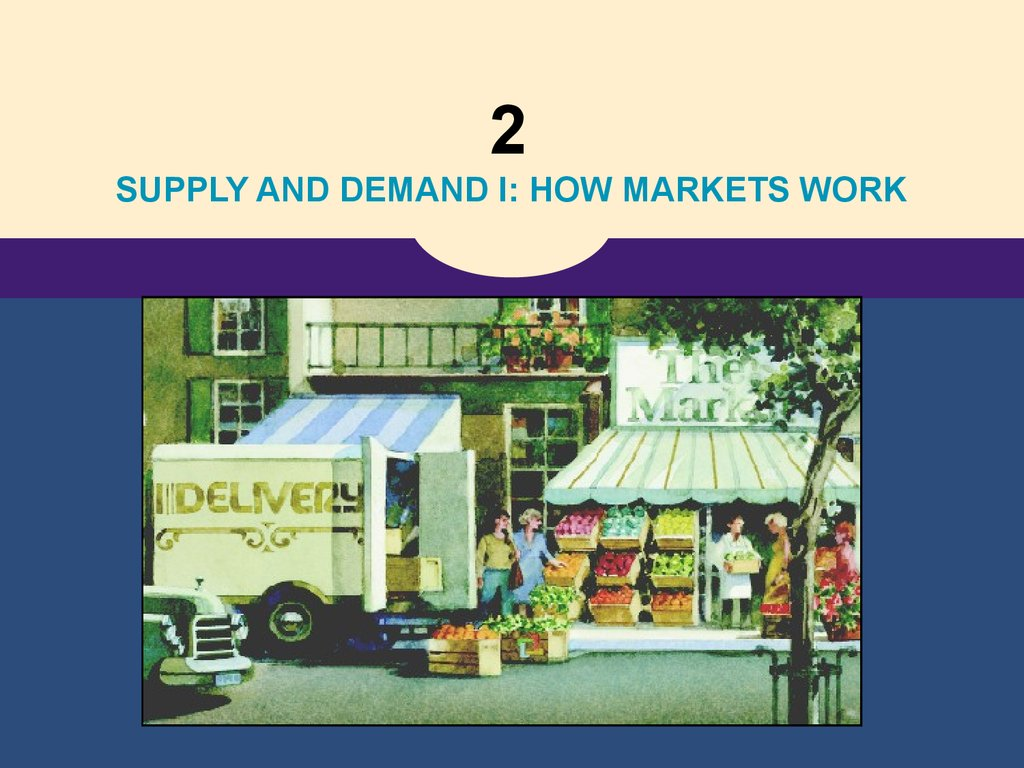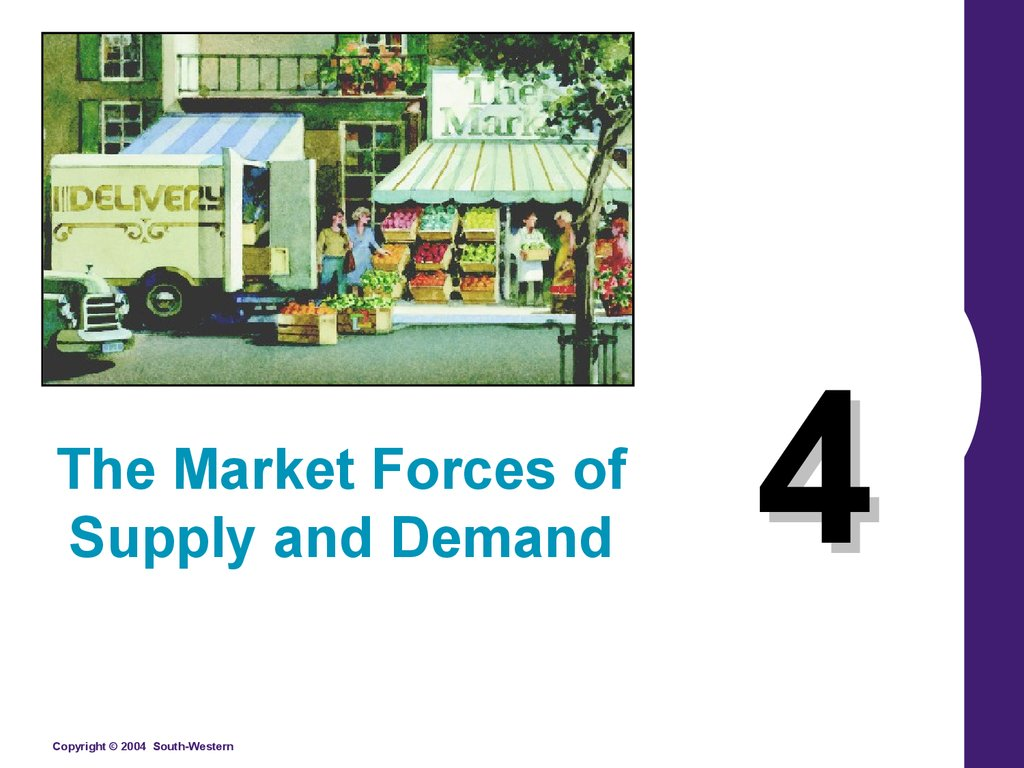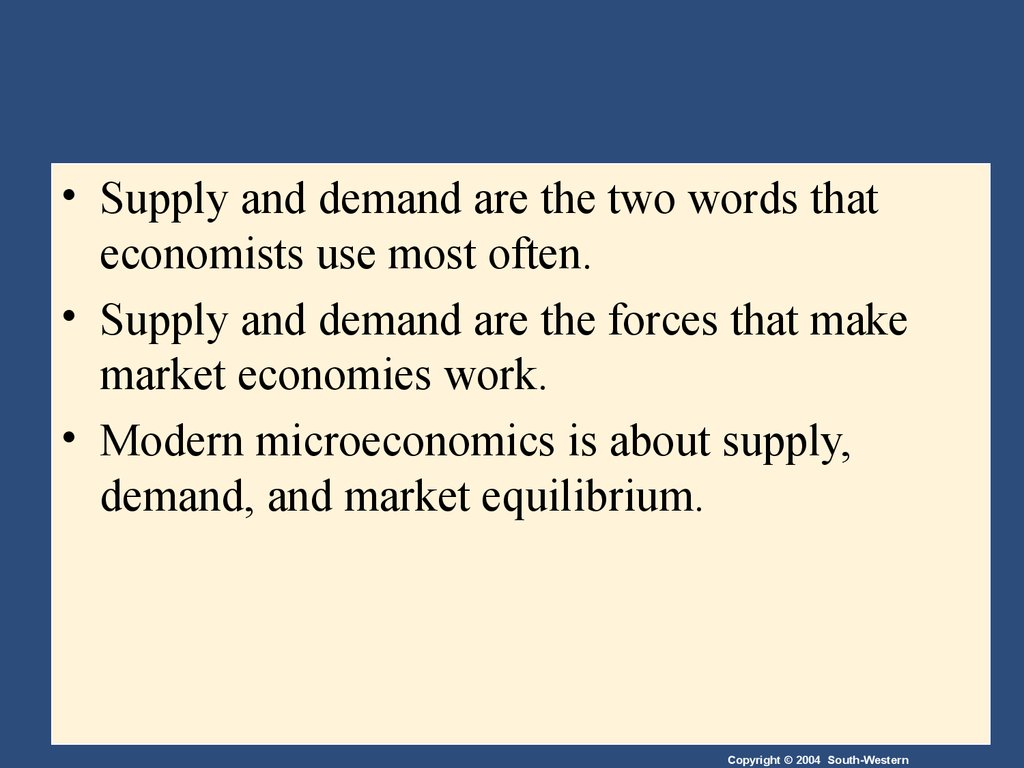# Supply and demand i: how markets work

## 1.

2
SUPPLY AND DEMAND I: HOW MARKETS WORK

## 2. 4

The Market Forces of
Supply and Demand
4

## 3.

• Supply and demand are the two words that
economists use most often.
• Supply and demand are the forces that make
market economies work.
• Modern microeconomics is about supply,
demand, and market equilibrium.

## 4. MARKETS AND COMPETITION

• A market is a group of buyers and sellers of a
particular good or service.
• The terms supply and demand refer to the
behavior of people . . . as they interact with one
another in markets.

## 5. MARKETS AND COMPETITION

• Sellers determine supply

## 6. Competitive Markets

• A competitive market is a market in which there
are many buyers and sellers so that each has a
negligible impact on the market price.

## 7. Competition: Perfect and Otherwise

• Perfect Competition
• Products are the same
• Numerous buyers and sellers so that each has no
influence over price
• Buyers and Sellers are price takers
• Monopoly
• One seller, and seller controls price

## 8. Competition: Perfect and Otherwise

• Oligopoly
• Few sellers
• Not always aggressive competition
• Monopolistic Competition
• Many sellers
• Slightly differentiated products
• Each seller may set price for its own product

## 9. DEMAND

• Quantity demanded is the amount of a good that
buyers are willing and able to purchase.
• Law of Demand
• The law of demand states that, other things equal,
the quantity demanded of a good falls when the
price of the good rises.

## 10. The Demand Curve: The Relationship between Price and Quantity Demanded

• Demand Schedule
• The demand schedule is a table that shows the
relationship between the price of the good and the
quantity demanded.

## 12. The Demand Curve: The Relationship between Price and Quantity Demanded

• Demand Curve
• The demand curve is a graph of the relationship
between the price of a good and the quantity
demanded.

## 13. Figure 1 Catherine’s Demand Schedule and Demand Curve

Price of
Ice-Cream Cone
\$3.00
2.50
1. A decrease
in price ...
2.00
1.50
1.00
0.50
0 1 2 3 4 5 6 7 8 9 10 11 12 Quantity of
Ice-Cream Cones
2. ... increases quantity
of cones demanded.

## 14. Market Demand versus Individual Demand

• Market demand refers to the sum of all
individual demands for a particular good or
service.
• Graphically, individual demand curves are
summed horizontally to obtain the market
demand curve.

## 15. Shifts in the Demand Curve

• Change in Quantity Demanded
• Movement along the demand curve.
• Caused by a change in the price of the product.

## 16. Changes in Quantity Demanded

Price of IceCream
Cones
B
\$2.00
A tax that raises the
price of ice-cream
cones results in a
movement along the
demand curve.
A
1.00
D
0
4
8
Quantity of Ice-Cream Cones

## 17. Shifts in the Demand Curve

Consumer income
Prices of related goods
Tastes
Expectations

## 18. Shifts in the Demand Curve

• Change in Demand
• A shift in the demand curve, either to the left or
right.
• Caused by any change that alters the quantity
demanded at every price.

Price of
Ice-Cream
Cone
Increase
in demand
Decrease
in demand
Demand curve, D3
0
Demand
curve, D1
Demand
curve, D2
Quantity of
Ice-Cream Cones

## 20. Shifts in the Demand Curve

• Consumer Income
• As income increases the demand for a normal good
will increase.
• As income increases the demand for an inferior
good will decrease.

## 21. Consumer Income Normal Good

Price of IceCream Cone
\$3.00
An increase
in income...
2.50
Increase
in demand
2.00
1.50
1.00
0.50
D1
0 1
2 3 4 5 6 7 8 9 10 11 12
D2
Quantity of
Ice-Cream
Cones

## 22. Consumer Income Inferior Good

Price of IceCream Cone
\$3.00
2.50
An increase
in income...
2.00
Decrease
in demand
1.50
1.00
0.50
D2
0 1
D1
2 3 4 5 6 7 8 9 10 11 12
Quantity of
Ice-Cream
Cones

## 23. Shifts in the Demand Curve

• Prices of Related Goods
• When a fall in the price of one good reduces the
demand for another good, the two goods are called
substitutes.
• When a fall in the price of one good increases the
demand for another good, the two goods are called
complements.

## 25. SUPPLY

• Quantity supplied is the amount of a good that
sellers are willing and able to sell.
• Law of Supply
• The law of supply states that, other things equal, the
quantity supplied of a good rises when the price of
the good rises.

## 26. The Supply Curve: The Relationship between Price and Quantity Supplied

• Supply Schedule
• The supply schedule is a table that shows the
relationship between the price of the good and the
quantity supplied.

## 28. The Supply Curve: The Relationship between Price and Quantity Supplied

• Supply Curve
• The supply curve is the graph of the relationship
between the price of a good and the quantity
supplied.

## 29. Figure 5 Ben’s Supply Schedule and Supply Curve

Price of
Ice-Cream
Cone
\$3.00
1. An
increase
in price ...
2.50
2.00
1.50
1.00
0.50
0
1 2
3
4
5
6
7
8
9 10 11 12 Quantity of
Ice-Cream Cones
2. ... increases quantity of cones supplied.

## 30. Market Supply versus Individual Supply

• Market supply refers to the sum of all
individual supplies for all sellers of a particular
good or service.
• Graphically, individual supply curves are
summed horizontally to obtain the market
supply curve.

## 31. Shifts in the Supply Curve

Input prices
Technology
Expectations
Number of sellers

## 32. Shifts in the Supply Curve

• Change in Quantity Supplied
• Movement along the supply curve.
• Caused by a change in anything that alters the
quantity supplied at each price.

## 33. Change in Quantity Supplied

Price of IceCream
Cone
S
C
\$3.00
A rise in the price
of ice cream
cones results in a
movement along
the supply curve.
A
1.00
0
1
5
Quantity of
Ice-Cream
Cones

## 34. Shifts in the Supply Curve

• Change in Supply
• A shift in the supply curve, either to the left or right.
• Caused by a change in a determinant other than
price.

Price of
Ice-Cream
Cone
Supply curve, S3
Decrease
in supply
Supply
curve, S1
Supply
curve, S2
Increase
in supply
0
Quantity of
Ice-Cream Cones

## 37. SUPPLY AND DEMAND TOGETHER

• Equilibrium refers to a situation in which the
price has reached the level where quantity
supplied equals quantity demanded.

## 38. SUPPLY AND DEMAND TOGETHER

• Equilibrium Price
• The price that balances quantity supplied and
quantity demanded.
• On a graph, it is the price at which the supply and
demand curves intersect.
• Equilibrium Quantity
• The quantity supplied and the quantity demanded at
the equilibrium price.
• On a graph it is the quantity at which the supply and
demand curves intersect.

## 39. SUPPLY AND DEMAND TOGETHER

Demand Schedule
Supply Schedule
At \$2.00, the quantity demanded
is equal to the quantity supplied!

## 40. Figure 8 The Equilibrium of Supply and Demand

Price of
Ice-Cream
Cone
Supply
Equilibrium
Equilibrium price
\$2.00
Equilibrium
quantity
0
1
2
3
4
5
6
7
8
Demand
9 10 11 12 13
Quantity of Ice-Cream Cones

## 41. Figure 9 Markets Not in Equilibrium

(a) Excess Supply
Price of
Ice-Cream
Cone
Supply
Surplus
\$2.50
2.00
Demand
0
4
Quantity
demanded
7
10
Quantity
supplied
Quantity of
Ice-Cream
Cones

## 42. Equilibrium

• Surplus
• When price > equilibrium price, then quantity
supplied > quantity demanded.
• There is excess supply or a surplus.
• Suppliers will lower the price to increase sales, thereby
moving toward equilibrium.

## 43. Equilibrium

• Shortage
• When price < equilibrium price, then quantity
demanded > the quantity supplied.
• There is excess demand or a shortage.
• Suppliers will raise the price due to too many buyers
chasing too few goods, thereby moving toward
equilibrium.

## 44. Figure 9 Markets Not in Equilibrium

(b) Excess Demand
Price of
Ice-Cream
Cone
Supply
\$2.00
1.50
Shortage
Demand
0
4
Quantity
supplied
7
10
Quantity of
Quantity
Ice-Cream
demanded
Cones

## 45. Equilibrium

• Law of supply and demand
• The claim that the price of any good adjusts to bring
the quantity supplied and the quantity demanded for
that good into balance.

## 46. Three Steps to Analyzing Changes in Equilibrium

• Decide whether the event shifts the supply or
demand curve (or both).
• Decide whether the curve(s) shift(s) to the left
or to the right.
• Use the supply-and-demand diagram to see how
the shift affects equilibrium price and quantity.

## 47. Figure 10 How an Increase in Demand Affects the Equilibrium

Price of
Ice-Cream
Cone
1. Hot weather increases
the demand for ice cream . . .
Supply
New equilibrium
\$2.50
2.00
2. . . . resulting
in a higher
price . . .
Initial
equilibrium
D
D
0
7
3. . . . and a higher
quantity sold.
10
Quantity of
Ice-Cream Cones

## 48. Three Steps to Analyzing Changes in Equilibrium

• Shifts in Curves versus Movements along
Curves
• A shift in the supply curve is called a change in
supply.
• A movement along a fixed supply curve is called a
change in quantity supplied.
• A shift in the demand curve is called a change in
demand.
• A movement along a fixed demand curve is called a
change in quantity demanded.

## 49. Figure 11 How a Decrease in Supply Affects the Equilibrium

Price of
Ice-Cream
Cone
S2
1. An increase in the
price of sugar reduces
the supply of ice cream. . .
S1
New
equilibrium
\$2.50
Initial equilibrium
2.00
2. . . . resulting
in a higher
price of ice
cream . . .
Demand
0
4
7
3. . . . and a lower
quantity sold.
Quantity of
Ice-Cream Cones

## 51. Summary

• Economists use the model of supply and
demand to analyze competitive markets.
• In a competitive market, there are many buyers
and sellers, each of whom has little or no
influence on the market price.

## 52. Summary

• The demand curve shows how the quantity of a
good depends upon the price.
• According to the law of demand, as the price of a
good falls, the quantity demanded rises. Therefore,
the demand curve slopes downward.
• In addition to price, other determinants of how
much consumers want to buy include income, the
prices of complements and substitutes, tastes,
expectations, and the number of buyers.
• If one of these factors changes, the demand curve
shifts.

## 53. Summary

• The supply curve shows how the quantity of a
good supplied depends upon the price.
• According to the law of supply, as the price of a
good rises, the quantity supplied rises. Therefore,
the supply curve slopes upward.
• In addition to price, other determinants of how
much producers want to sell include input prices,
technology, expectations, and the number of sellers.
• If one of these factors changes, the supply curve
shifts.

## 54. Summary

• Market equilibrium is determined by the
intersection of the supply and demand curves.
• At the equilibrium price, the quantity demanded
equals the quantity supplied.
• The behavior of buyers and sellers naturally
drives markets toward their equilibrium.